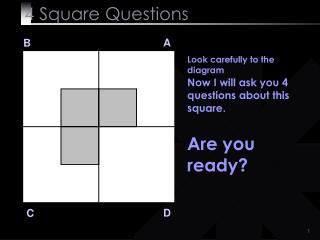# Solve - if you are not too busy - PowerPoint PPT PresentationDownload PresentationSolve - if you are not too busy

Solve - if you are not too busy
Download Presentation## Solve - if you are not too busy

- - - - - - - - - - - - - - - - - - - - - - - - - - - E N D - - - - - - - - - - - - - - - - - - - - - - - - - - -
##### Presentation Transcript

2. 4 SquareQuestions A B Q 1 Q 1 Divide the white area in square A into two equal pieces. Easy!! Isn`t it? D C

3. 4 SquareQuestions A B Q 1 Q 1 Divide the white area in square A into two equal pieces. Here is the answer! D C

4. 4 SquareQuestions A B Q 1 Q 1 Divide the white area in square A into two equal pieces. Of course you solved it! :) D C

5. 4 SquareQuestions A B Q 2 Q2 Divide the white area in square B into three equal pieces. Come on it is not soo difficult! D C

6. 4 SquareQuestions A B Q 2 Q 2 Divide the white area in square B into three equal pieces. Here is the answer! D C

7. 4 SquareQuestions A B Q 2 Q 2 Divide the white area in square B into three equal pieces. You knew the answer anyways or?? :)) D C

8. 4 SquareQuestions A B OK!!! D C

9. 4 SquareQuestions A B Q 3 Q 3 Divide the white area in square C into four equal pieces. Very difficult?? That`s right! D C

10. 4 SquareQuestions A B Q 3 Q 3 Divide the white area in square C into four equal pieces. You haven´t found it yet??? D C

11. 4 SquareQuestions A B Q 3 Q 3 Divide the white area in square C into four equal pieces. Come on! You can do it!! D C

12. 4 SquareQuestions A B Q 3 Q 3 Divide the white area in square C into four equal pieces. Take your time. Click If you want to see the solution! D C

13. 4 SquareQuestions A B Q 3 Q 3 Divide the white area in square C into four equal pieces. Here is the solution! D C

14. 4 SquareQuestions A B Q 3 Q 3 Divide the white area in square C into four equal pieces. Could you solve it? :))) D C

15. 4 SquareQuestions A B Be ready here comes the last Question! D C

16. 4 SquareQuestions A B Q 4 Q 4 Divide the area D into seven equal pieces. World record is 7 seconds!! D C

17. 4 SquareQuestions A B Q 4 Q 4 Divide the area D into seven equal pieces. World record is 7 seconds!! D C

18. 4 SquareQuestions A B Q 4 Q4 Divide the area D into seven equal pieces. Time is up! D C

19. 4 SquareQuestions A B Q 4 Q 4 Divide the area D into seven equal pieces. Any idea?? D C

20. 4 SquareQuestions A B Q 4 Q 4 Divide the area D into seven equal pieces. I can wait!! Click when you are bored! D C

21. 4 SquareQuestions A B Q 4 Q 4 Divide the area D into seven equal pieces. Here is the answer! D C

22. 4 SquareQuestions A B Q 4 Q 4 Divide the area D into seven equal pieces. Was it really that difficult? D C

23. 4 SquareQuestions A B Q 4 Q 4 Divide the area D into seven equal pieces. It was just to see how our minds can be conditioned! :)))) D C

24. 4 SquareQuestions • Lesson learnt : • Most of the times, our mind gets conditioned so much by the circumstances that - we can not see the obvious. • When we go to a specialist doctor with a simple headache, diagnosis starts from migraine, upwards! • We assume that things are so complicated and we get entrapped in the ghost of it’s thoughts • We carry on the same syndrome to our relationships and make them complicated

25. 4 SquareQuestions BE SIMPLE IN LIFE !! GREATNESS OF THIS MAN WAS HIS SIMPLICITY

26. 4 SquareQuestions HAVE A NICE DAY!!